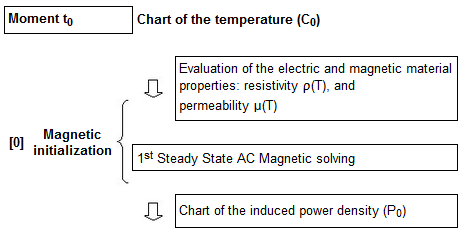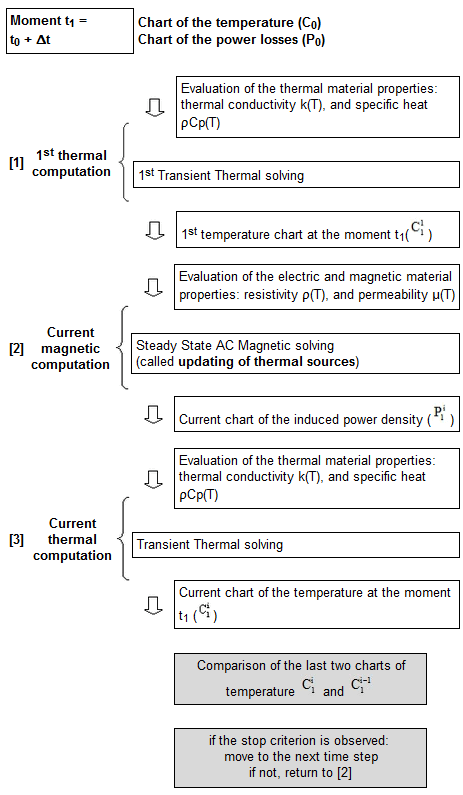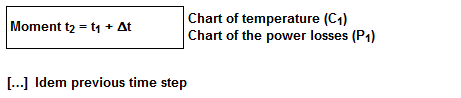# Principle of coupling

## The coupling …

The coupling proposed in Flux, corresponding to the step by step in time domain method, is a weak coupling: the thermal and the magnetic problems are solved successively.

The systems of equations of the two problems are coupled by means of certain terms:

• the temperature resulting from the solving of the thermal problem is a quantity involved in the system of magnetic equations by means of the temperature dependence of the electromagnetic properties of the part to be heated: magnetic permeability and electric conductivity;
• the induced power losses resulting from the solving of the magnetic problem is the term corresponding to the heating source of the system of thermal equations

## Hypotheses for the computation

The main computation hypotheses are the following:

• the electric currents are alternating and they can be considered as having a sinusoidal time dependence;
• the variation with respect to the temperature of the physical properties is slow and continuous;
• the magnetic state of the device can be considered as harmonic

## Principle: presentation

The magneto thermal coupling proposed in Flux is a coupling between the following two applications:

• the Steady State AC Magnetic application (1) which allows the computation of the active power dissipated by Joule effect in the heated components of the studied device;
• the Transient Thermal application (2) which allows the study of the temperature evolution in the heated components of the device.

The power dissipated by Joule effect of induced currents, computed with application 1, is introduced as source of the thermal problem in application 2.

The temperature computed with application 2 is considered in application 1 for the evaluation of the material characteristics.

Indeed, at each time step, a local linking* of Thermal and Steady State AC Magnetic computations is achieved, until the convergence of the thermal problem is achieved, i.e. until the steadiness of the temperature field corresponding to the analyzed time step is achieved.

* The detail of the linking is shown in the following section.

## Principle: details

The principle of the magneto thermal coupling in Flux is detailed below.